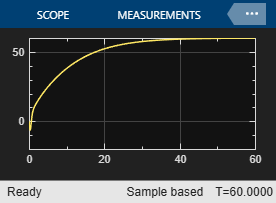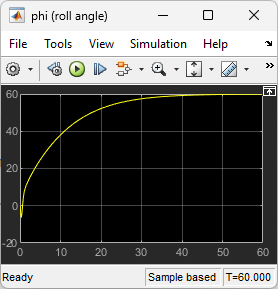# Design LQR Servo Controller in Simulink

This example shows the design of an LQR servo controller in Simulink® using an aircraft autopilot application.

Open the model for the

`open_system("lqrpilot")`In this model:

• The `Linearized Dynamics` block contains the linearized airframe.

• `sf_aerodyn` is an S-Function block that contains the nonlinear equations for $\left(\theta ,\varphi \right)=\left(0,{15}^{\circ }\right)$.

• The error signal between $\varphi$ and the ${\varphi }_{\mathrm{ref}}$ is passed through an integrator, which helps drive error to zero.

Opening the model also loads the `lqrpilotData` MAT file, which contains the following data.

• State equation matrices `A` and `B`

• Linearized state matrix `A15`

• Final LQG gain matrix `K_lqr`

### Aircraft State-Space Equations

The equation is the standard state equation of a state-space system.

`$\stackrel{˙}{\mathit{x}}=\mathit{A}\mathit{x}+\mathit{B}\mathit{u}$`

For the aircraft system, the state vector is as follows.

`$\mathit{x}={\left[\mathit{u},\mathit{v},\mathit{w},\mathit{p},\mathit{q},\mathit{r},\theta ,\varphi \right]}^{\mathit{T}}$`

The variables $\mathit{u}$, $\mathit{v}$, and $\mathit{w}$ are the three velocities with respect to the body frame, as shown in the following figure.The variables $\varphi$ and $\theta$ are roll and pitch. $\mathit{p}$, $\mathit{q}$, and $\mathit{r}$ are the roll, pitch, and yaw rates, respectively.

The airframe dynamics are nonlinear. The following equation shows the nonlinear components added to the state space equation, where $\mathit{g}$ is the acceleration due to gravity.

`$\stackrel{˙}{\mathit{x}}=\mathit{Ax}+\mathit{Bu}+\left[\begin{array}{c}-\mathit{g}\cdot \mathrm{sin}\theta \\ \mathit{g}\cdot \mathrm{cos}\theta \cdot \mathrm{sin}\varphi \\ \mathit{g}\cdot \mathrm{cos}\theta \cdot \mathrm{cos}\varphi \\ 0\\ 0\\ 0\\ \mathit{q}\cdot \mathrm{cos}\varphi -\mathit{r}\cdot \mathrm{sin}\varphi \\ \left(\mathit{q}\cdot \mathrm{sin}\varphi +\mathit{r}\cdot \mathrm{cos}\varphi \right)\cdot \mathrm{tan}\theta \end{array}\right]$`

### Trimming

For LQG design purposes, the nonlinear dynamics are trimmed at $\varphi ={15}^{\circ }$ and $\mathit{p}$, $\mathit{q}$, $\mathit{r}$, and $\theta$ set to zero. Since $\mathit{u}$, $\mathit{v}$, and $\mathit{w}$ do not affect into the nonlinear term in the preceding equation, the result is a model linearized around $\left(\theta ,\varphi \right)=\left(0,{15}^{\circ }\right)$ with all remaining states set to zero.

The `lqrdes` script shows how to compute the linearized model `A15` at this trimmed operating point.

### Problem Definition

The goal for the design is to perform a steady coordinated turn, as shown in this figure.To achieve this goal, you must design a controller that commands a steady turn by going through a 60° roll. In addition, assume that the pitch angle $\theta$ must stay as close to zero as possible.

### Results

The `lqrdes` script shows how to calculate the LQG gain matrix `K_lqr`.

In the `lqrpilot` model, ensure that the switch block is configured to select the output of the Nonlinear Dynamics block.

Run the model.

`sim("lqrpilot")`

View the response of the roll $\varphi$ to a step-change of 60°. The system tracks the commanded roll within about 60 seconds.

`open_system("lqrpilot/phi (roll angle)")`View the pitch angle $\theta$. The controller was able to keep the pitch angle relatively small.

`open_system("lqrpilot/theta (pitch angle)")`Finally, view the control inputs.

`open_system("lqrpilot/Control Inputs")`You can adjust the Q and R values in the `lqrdes` script to try different potential designs. Also, you can compare simulations of the linear and nonlinear system dynamics to see the effects of the nonlinearities on the system performance.